# (a) For the double integral pin2 (In 2)2-y I = ef+y* dx dy. i. Sketch the...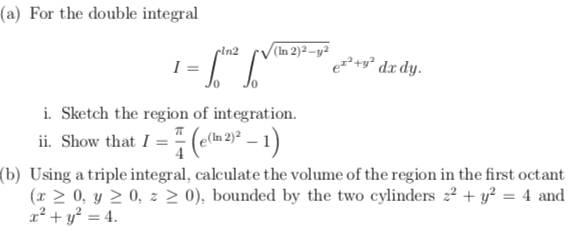(a) For the double integral pin2 (In 2)2-y I = ef+y* dx dy. i. Sketch the region of integration. ii. Show that I = (extu 2) (b) Using a triple integral, calculate the volume of the region in the first octant (x > 0, y > 0, z > 0), bounded by the two cylinders z2 + y2 = 4 and x² + y2 = 4.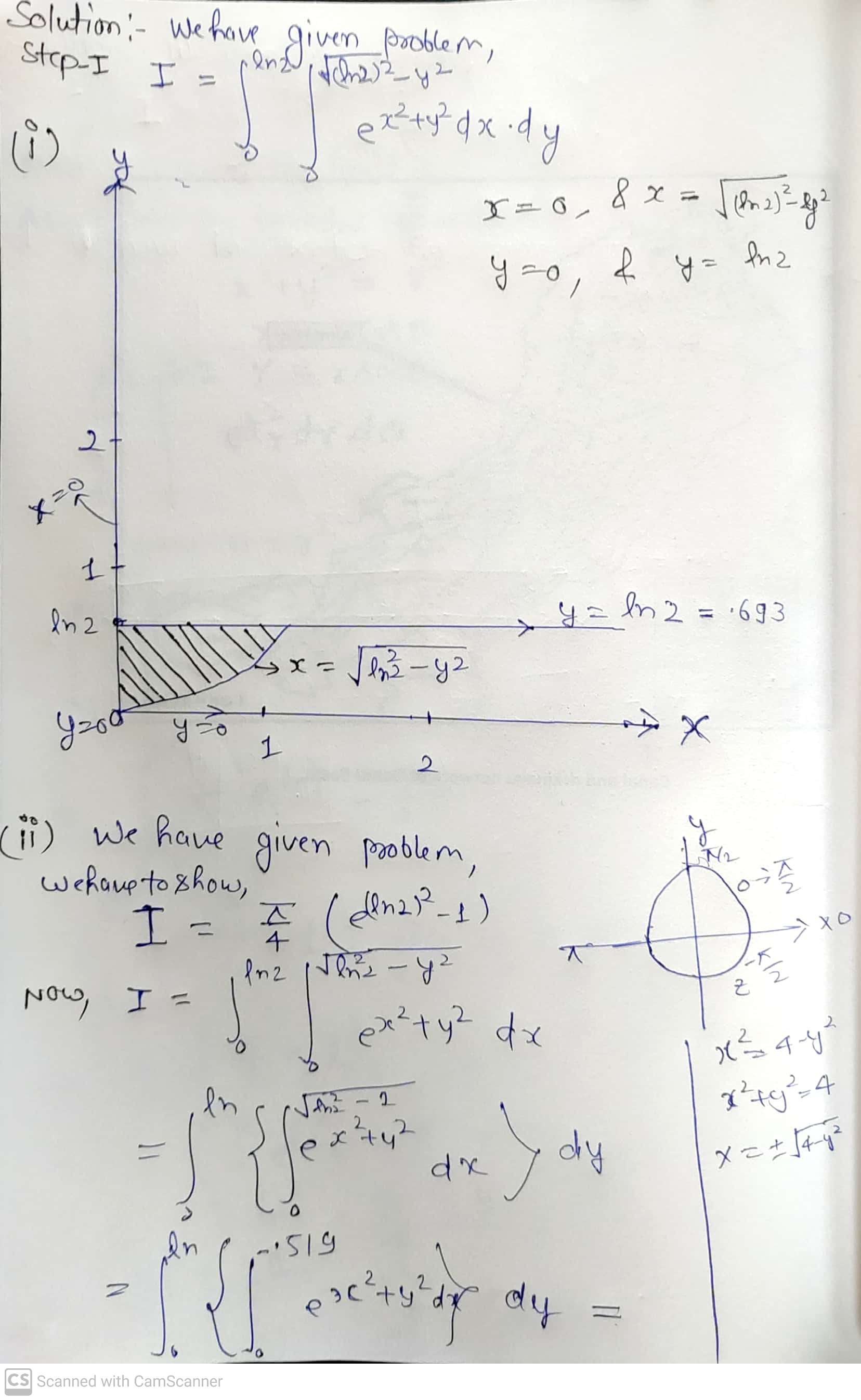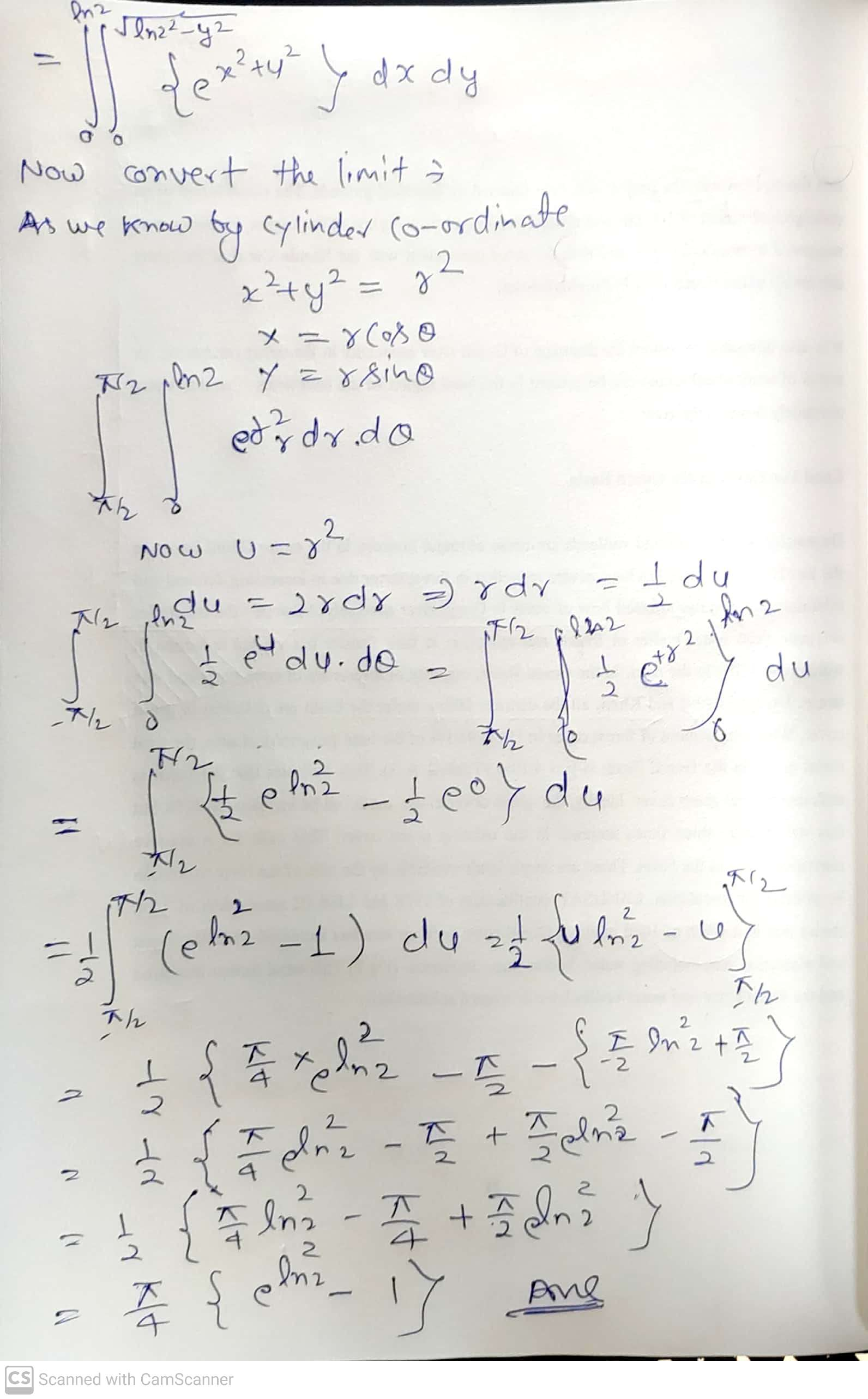##### Add Answer of: (a) For the double integral pin2 (In 2)2-y I = ef+y* dx dy. i. Sketch the...
Similar Homework Help Questions
• ### 1 point) Integratef(x, y, z) 6xz over the region in the first octant (x,y, z 0) above the parabol...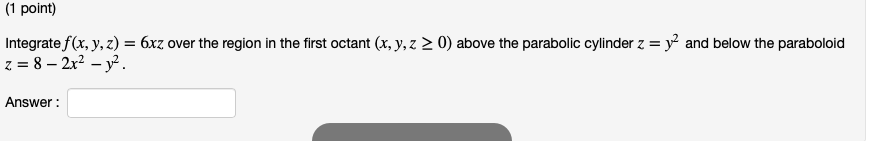Please try helping with all three questions.......please 1 point) Integratef(x, y, z) 6xz over the region in the first octant (x,y, z 0) above the parabolic cylinder z = y2 and below the paraboloid Answer Find the volume of the solid in R3 bounded by y-x2 , x-уг, z-x + y + 24, and Z-0. Consider the triple integral fsPw xyz2 dV, where W is the region bounded by Write the triple integral as an iterated integral in the order...

• ### 2 147 a. Evaluate the triple integral (convert to oylindrical)12I, J xz dz dx dy b. Find the mome...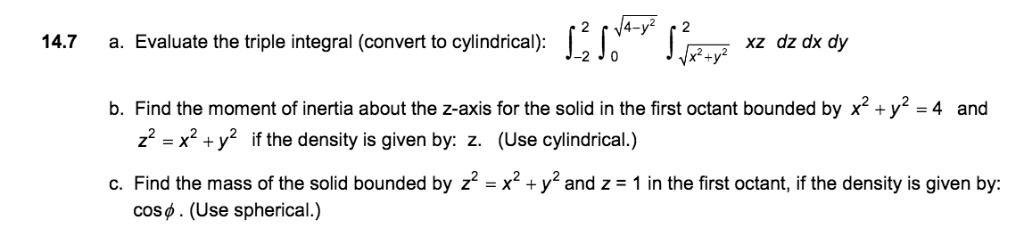2 147 a. Evaluate the triple integral (convert to oylindrical)12I, J xz dz dx dy b. Find the moment of inertia about the z-axis for the solid in the first octant bounded by x2+y2 -4 and z2-x2 + y2 if the density is given by: z. (Use cylindrical.) c. Find the mass of the solid bounded by z2 -x2 +y2 and z 1 in the first octant, if the density is given by: cos. (Use spherical.) 2 147 a. Evaluate...

• ### Using a triple integral, calculate the volume of the region in the first octant (x >...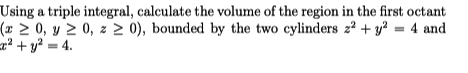Using a triple integral, calculate the volume of the region in the first octant (x > 0, y 20, z > 0), bounded by the two cylinders z2 + y2 = 4 and c? + y2 = 4.

• ### x=7 dy dz dx = x Z=0 The given triple integral sss =49-x² Y = 7-8...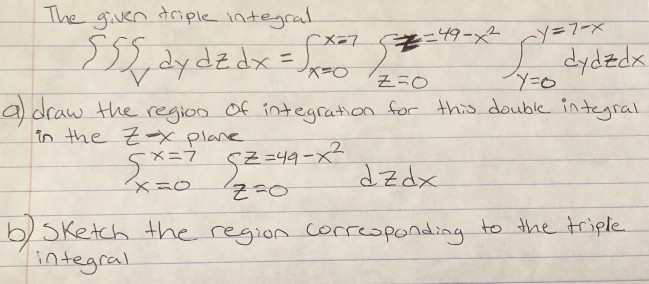x=7 dy dz dx = x Z=0 The given triple integral sss =49-x² Y = 7-8 L dydzdx a) draw the region of integration for this double integral in the Zx plane 5*=7 62-4 dzdx z=0 b) Sketch the region corresponding to the triple integral SZ=49-x²

• ### Find the volume of the given solid region in the first octant bounded by the plane 2x + 2y + 4z4 and the coordinate planes, using triple integrals 0.0(020 Complete the triple integral below used to f...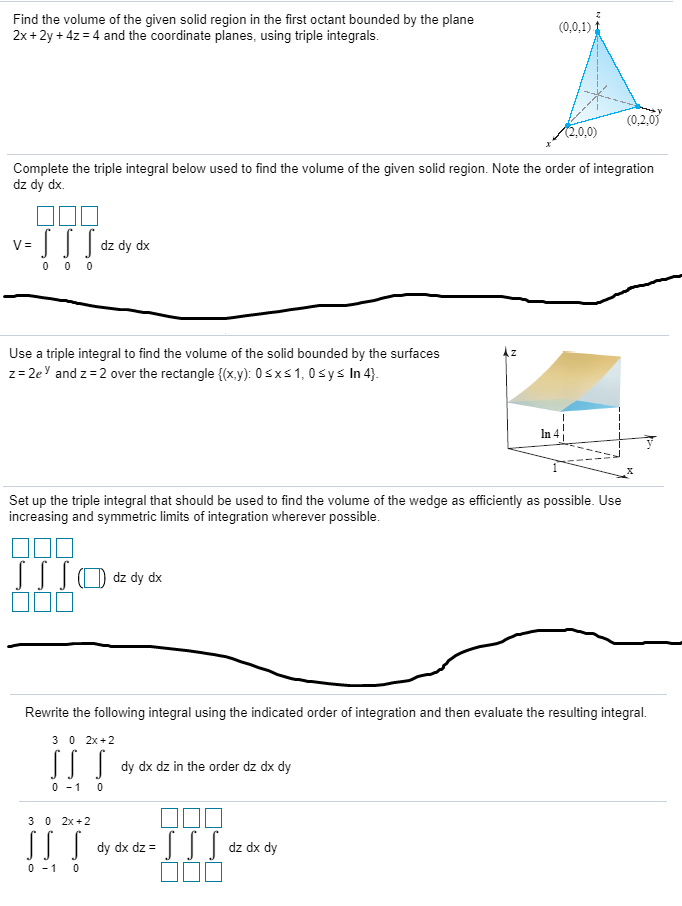Find the volume of the given solid region in the first octant bounded by the plane 2x + 2y + 4z4 and the coordinate planes, using triple integrals 0.0(020 Complete the triple integral below used to find the volume of the given solid region. Note the order of integration dz dy dx. dz dy dx Use a triple integral to find the volume of the solid bounded by the surfaces z-2e and z 2 over the rectangle (x.y): 0 sxs1,...

• ### 8. Sketch the region of integration and evaluate the integral re dx dy, where G is the region bounded by 0,1, -o,y- 8. Sketch the region of integration and evaluate the integral re dx dy, where...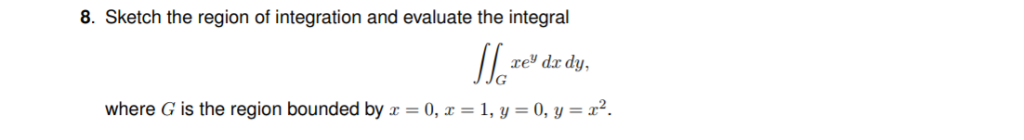8. Sketch the region of integration and evaluate the integral re dx dy, where G is the region bounded by 0,1, -o,y- 8. Sketch the region of integration and evaluate the integral re dx dy, where G is the region bounded by 0,1, -o,y-

• ### please show complete work 25) Use a triple integral in the coordinate system of your choice to find the volume of the s...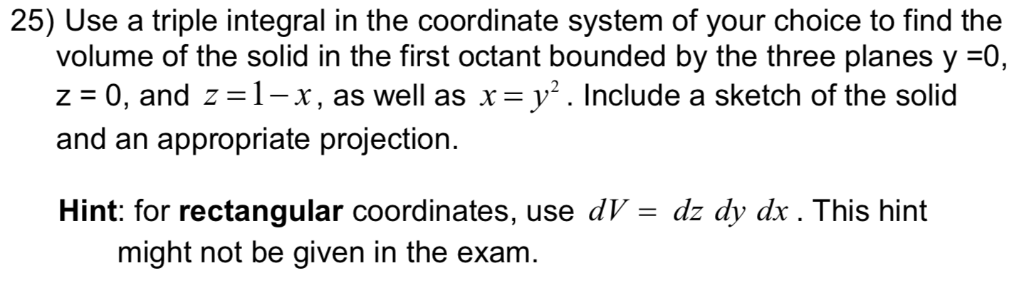please show complete work 25) Use a triple integral in the coordinate system of your choice to find the volume of the solid in the first octant bounded by the three planes y =0 z 0, and z 1-x x y2. Include a sketch of the solid as well as appropriate projection and an Hint: for rectangular coordinates, use dV might not be given in the exam dz dy dx. This hint 25) Use a triple integral in the coordinate...

• ### 6. (4 pts) Consider the double integral∫R(x2+y)dA=∫10∫y−y(x2+y)dxdy+∫√21∫√2−y2−√2−y2(x2+y)dxdy.(a) Sketch the region of integration R in Figure 3.(b)...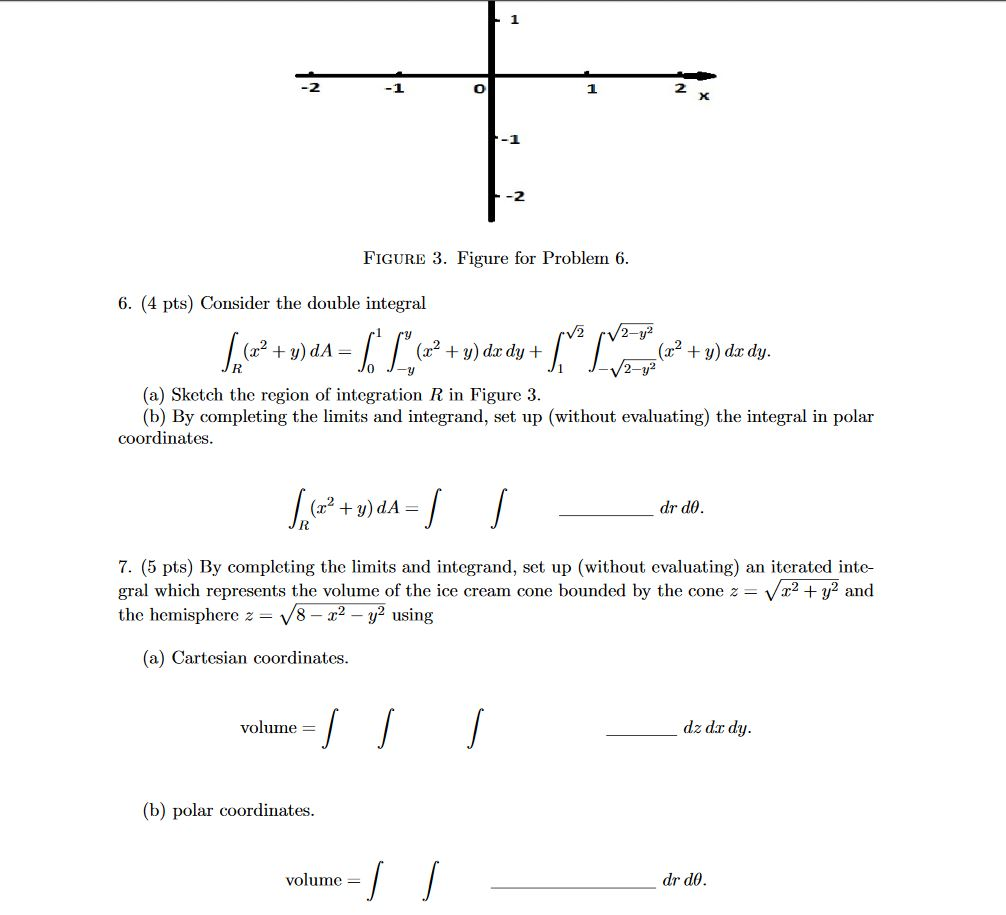6. (4 pts) Consider the double integral∫R(x2+y)dA=∫10∫y−y(x2+y)dxdy+∫√21∫√2−y2−√2−y2(x2+y)dxdy.(a) Sketch the region of integration R in Figure 3.(b) By completing the limits and integrand, set up (without evaluating) the integral in polar coordinates.∫R(x2+y)dA=∫∫drdθ.7. (5 pts) By completing the limits and integrand, set up (without evaluating) an iterated inte-gral which represents the volume of the ice cream cone bounded by the cone z=√x2+y2andthe hemisphere z=√8−x2−y2using(a) Cartesian coordinates.volume =∫∫∫dz dxdy.(b) polar coordinates.volume =∫∫drdθ. -1 -2 FIGURE 3. Figure for Problem 6. 6. (4 pts)...

• ### 6. (4 pts) Consider the double integral∫R(x2+y)dA=∫10∫y−y(x2+y)dxdy+∫√21∫√2−y2−√2−y2(x2+y)dxdy.(a) Sketch the region of integrationRin Figure 3.(b) By completing...6. (4 pts) Consider the double integral∫R(x2+y)dA=∫10∫y−y(x2+y)dxdy+∫√21∫√2−y2−√2−y2(x2+y)dxdy.(a) Sketch the region of integrationRin Figure 3.(b) By completing the limits and integrand, set up (without evaluating) the integral in polar coordinates. -1 -2 FIGURE 3. Figure for Problem 6. 6. (4 pts) Consider the double integral V2 /2-y² + = (x2 + y) dx dy + + y) do dy. 2-y2 (a) Sketch the region of integration R in Figure 3. (b) By completing the limits and integrand, set up (without evaluating)...

• ### Please Answer the Following Questions (SHOW ALL WORK) 1. 2. 3. 4. Write an iterated integral...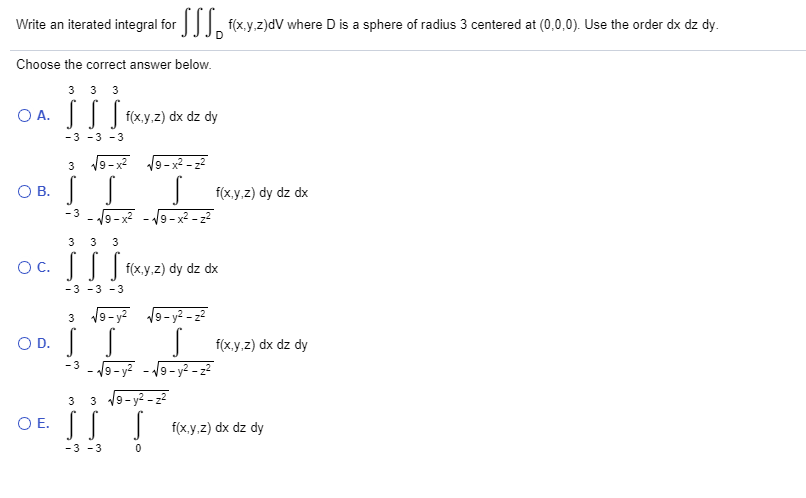Please Answer the Following Questions (SHOW ALL WORK) 1. 2. 3. 4. Write an iterated integral for SSSo flexy.z)dV where D is a sphere of radius 3 centered at (0,0,0). Use the order dx dz dy. Choose the correct answer below. 3 3 3 OA. S S f(x,y,z) dx dz dy -3 -3 -3 3 OB. S 19-x2 19-32-22 s f(x,y,z) dy dz dx 19-x2 - 19-2-22 s -3 3 3 3 oc. S S [ f(x,y,z) dy dz dx...

Free Homework App